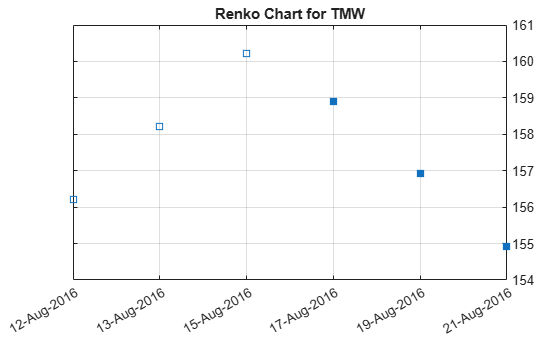# renko

## Syntax

``renko(Data)``
``renko(Data,Threshold,)``
``h = renko(ax,___)``

## Description

example

````renko(Data)` plots a Renko chart from a series of prices of a security.```

example

````renko(Data,Threshold,)` adds an optional argument for `Threshold`. ```

example

````h = renko(ax,___)` adds an optional argument for `ax`. ```

## Examples

collapse all

Load the file `SimulatedStock.mat`, which provides a timetable (`TMW`) for financial data for TMW stock. This example shows how to plot a Renko chart for the most recent 21 days. Note that the variable name of asset price is renamed to `'Price'` (case insensitive).

```load SimulatedStock.mat TMW.Properties.VariableNames{'Close'} = 'Price'; renko(TMW(end-8:end,:),2) title('Renko Chart for TMW')```## Input Arguments

collapse all

Data for a series of prices, specified as a matrix, table, or timetable. Timetables and tables with `M` rows must contain a variable named `'Price'` (case insensitive).

Data Types: `double` | `table` | `timetable`

Least price change value when adding a new box, specified as a scalar positive numeric value.

Data Types: `double`

(Optional) Valid axis object, specified as an axes object. The renko plot is created in the axes specified by `ax` instead of in the current axes (`ax = gca`). The option `ax` can precede any of the input argument combinations.

Data Types: `object`

## Output Arguments

collapse all

Graphic handle of the figure, returned as a handle object.

## Version History

Introduced in R2008a

expand all